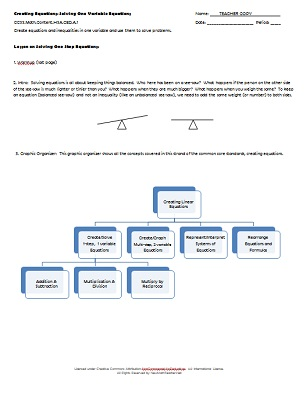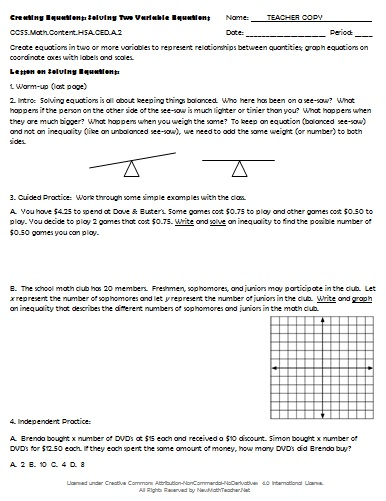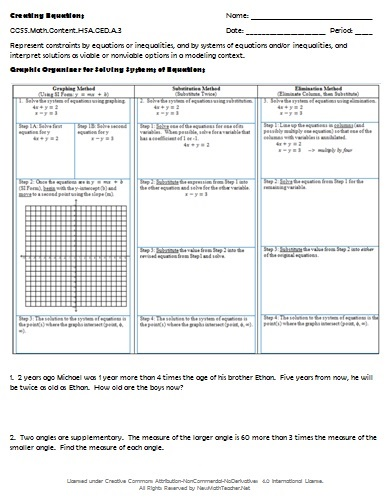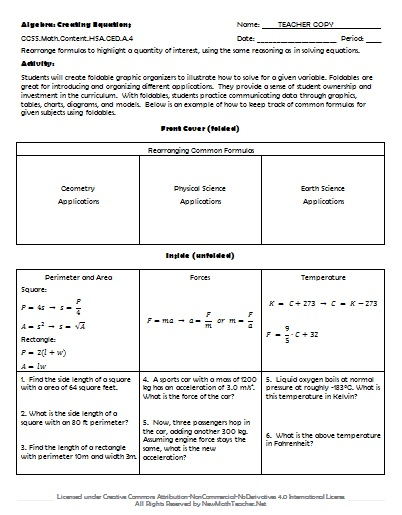# Engaging Math Lessons.

Please browse our selection of mathematics lessons sorted by subject and current common core standards for improving math achievement for every student.

Below are a comprehensive set of lesson plans and worksheets for Algebra 1 that correspond to the given Common Core concept and help students solve real-world problems. Lesson previews provide a quick glimpse at the provided materials.

• ### Concept:

Creating Equations
• Common Core Standard:CCSS.Math.Content.HSA.CED.A.1
• Description: Create equations and inequalities in one variable and use them to solve problems.• ### Concept:

Creating Equations
• Common Core Standard:CCSS.Math.Content.HSA.CED.A.2
• Description: Create equations in two or more variables to represent relationships between quantities; graph equations on coordinate axes with labels and scales.• ### Concept:

Creating Equations
• Common Core Standard:CCSS.Math.Content.HSA.CED.A.3
• Description: Represent constraints by equations or inequalities, and by systems of equations and/or inequalities, and interpret solutions as viable or nonviable options in a modeling context.• ### Concept:

Creating Equations
• Common Core Standard:CCSS.Math.Content.HSA.CED.A.4
• Description: Rearrange formulas to highlight a quantity of interest, using the same reasoning as in solving equations.